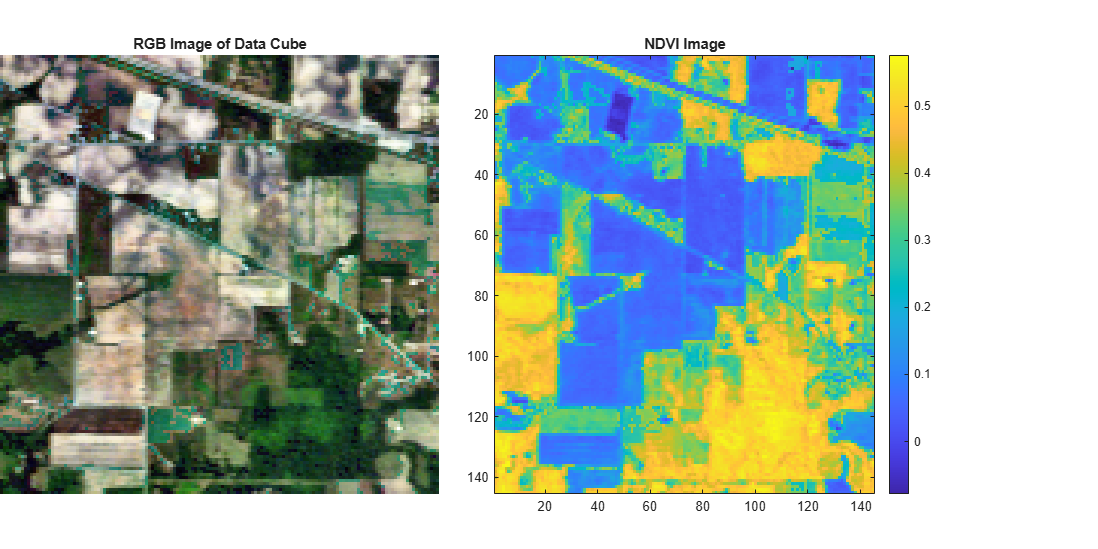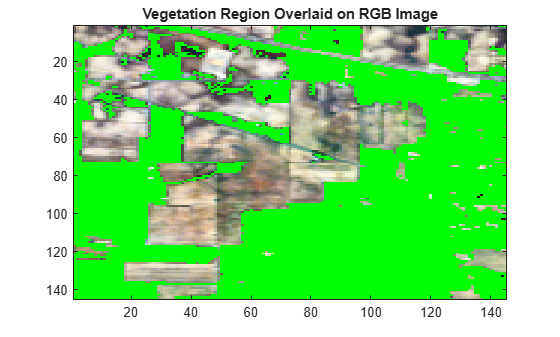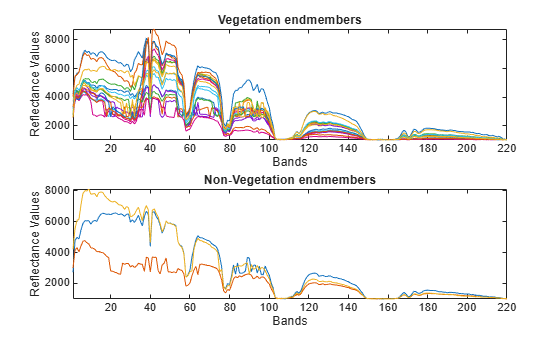# ndvi

Normalized difference vegetation index

## Syntax

``output = ndvi(hcube)``
``output = ndvi(hcube,'BlockSize',blocksize)``

## Description

example

````output = ndvi(hcube)` computes the normalized difference vegetation index (NDVI) value for each pixel in the data cube and returns an NDVI image. The NDVI image displays the vegetation cover regions of the input hyperspectral data. The function computes the NDVI value using the red (R) band and the near-infra red (NIR) band images in the data cube. The `ndvi` function uses the 670 nm and 800 nm band reflectance values for the red and NIR band images respectively.```
````output = ndvi(hcube,'BlockSize',blocksize)` specifies the block size for block processing of the hyperspectral data cube by using the name-value pair argument `'BlockSize'`.The function divides the input image into distinct blocks, processes each block, and then concatenates the processed output of each block to form the output matrix. Hyperspectral images are multi-dimensional data sets that can be too large to fit in system memory in their entirety. This can cause the system to run out of memory while running the `ndvi` function. If you encounter such an issue, perform block processing by using this syntax.For example, `ndvi(hcube,'BlockSize',[50 50])` divides the input image into non-overlapping blocks of size 50-by-50 and then computes the NDVI values for pixels in each block. NoteTo perform block processing by specifying the `'BlockSize'` name-value pair argument, you must have MATLAB® R2021a or a later release. NoteThis function requires the Image Processing Toolbox™ Hyperspectral Imaging Library. You can install the Image Processing Toolbox Hyperspectral Imaging Library from Add-On Explorer. For more information about installing add-ons, see Get and Manage Add-Ons. ```

## Examples

collapse all

Read hyperspectral data into the workspace.

`hcube = hypercube('indian_pines.dat');`

Compute the NDVI value for each pixel in the data cube.

`ndviImg = ndvi(hcube);`

Estimate a contrast-stretched RGB image from the original data cube by using the `colorize` function.

`rgbImg = colorize(hcube,'Method','RGB','ContrastStretching',true);`

Display the original and the NDVI image.

```fig = figure('Position',[0 0 1200 600]); axes1 = axes('Parent',fig,'Position',[0 0.1 0.4 0.8]); imshow(rgbImg,'Parent',axes1) title('RGB Image of Data Cube') axes2 = axes('Parent',fig,'Position',[0.45 0.1 0.4 0.8]); imagesc(ndviImg,'Parent',axes2) colorbar title('NDVI Image')```Vegetation regions typically have NDVI values from 0.2 and 0.8. NDVI values less than or equal to 0.2 indicate the absence of vegetation. Perform thresholding of NDVI image to segment the vegetation regions. Specify the threshold value.

`threshold = 0.2;`

Generate a binary image with a intensity value `1` for pixels with a score greater than or equal to the specified threshold. All other pixels have a value 0. The regions in the binary image with a value of `1` correspond to the vegetation regions in the data cube with NDVI values greater than the threshold.

`bw = ndviImg > threshold;`

Overlay the binary image on to the RGB image and display the overlaid image.

```overlayImg = imoverlay(rgbImg,bw,[0 1 0]); figure imagesc(overlayImg) title('Vegetation Region Overlaid on RGB Image')```Compute the vegetation cover based on the total number of pixels in a spectral band and the number of pixels with an NDVI value greater than 0.2.

```numVeg = find(bw == 1); imgSize = size(hcube.DataCube,1)*size(hcube.DataCube,2); vegetationCover = length(numVeg)/imgSize```
```vegetationCover = 0.5696 ```

This example shows you how to:

• Use 2-D spectral data as a hypercube for the hyperspectral functions.

• Separate vegetation and non-vegetation spectra by using `ndvi` function.

Load 2-D Spectral Data

Load 2-D spectral data containing 20 endmembers of the Indian Pines data set into the workspace.

`load("indian_pines_endmembers_20.mat")`

Load the wavelength values for each band of the Indian Pines data set into the workspace.

`load("indian_pines_wavelength.mat")`

Prepare Test Data to Use for Hyperspectral Functions

Reshape the 2-D spectral data into a 3-D volume data using the `reshape` function.

```[numSpectra,spectralDim] = size(endmembers); dataCube = reshape(endmembers,[numSpectra 1 spectralDim]);```

Create a 3-D `hypercube` object, with a singleton dimension, by specifying the 3-D volume data `dataCube` and wavelength information `wavelength` to the `hypercube` function.

`hCube = hypercube(dataCube,wavelength);`

Compute NDVI to Separate Vegetation and Non-Vegetation Spectra

Compute the NDVI value for each spectrum in the hypercube object.

`ndviVal = ndvi(hCube);`

Vegetation spectra typically have NDVI values greater than zero and non-vegetation spectra typically have NDVI values less than zero. Perform thresholding to separate the vegetation and non-vegetation spectra.

`index = ndviVal > 0;`

Plot the vegetation and non-vegetation endmembers.

```subplot(2,1,1) plot(endmembers(index,:)') title("Vegetation endmembers") xlabel("Bands") ylabel("Reflectance Values") axis tight subplot(2,1,2) plot(endmembers(~index,:)') title("Non-Vegetation endmembers") xlabel("Bands") ylabel("Reflectance Values") axis tight```## Input Arguments

collapse all

Input hyperspectral data, specified as a `hypercube` object. The functions reads the hyperspectral data cube from the `DataCube` property of the object and then computes the NDVI value of each pixel.

Size of the data blocks, specified as a 2-element vector of positive integers. The elements of the vector correspond to the number of rows and columns in each block, respectively. The size of the data blocks must be less than the size of the input image. Dividing the hyperspectral images into smaller blocks enables you process large data sets without running out of memory.

• If the `blocksize` value is too small, the memory usage of the function reduces at the cost of increased execution time.

• If the `blocksize` value is large or equal to the input image size, the execution time reduces at the cost of increased memory usage.

Example: `'BlockSize',[20 20]` specifies the size of each data block as 20-by-20.

## Output Arguments

collapse all

Output NDVI image, returned as a matrix of size M-by-N. M and N are spatial dimensions of the input data cube. If the data type of the input data cube is `double`, the output data type is also `double`. Otherwise, the output data type is `single`.

The function computes the NDVI value for each pixel as

`$NDVI=\text{\hspace{0.17em}}\text{\hspace{0.17em}}\frac{NIR-R}{NIR+R},$`

The values are in the range [-1, 1]. A value close to 1 indicates healthy vegetation, 0 indicates unhealthy vegetation, and -1 indicates no vegetation.

Data Types: `single` | `double`

 Haboudane, D. “Hyperspectral Vegetation Indices and Novel Algorithms for Predicting Green LAI of Crop Canopies: Modeling and Validation in the Context of Precision Agriculture.” Remote Sensing of Environment 90, no. 3 (April 15, 2004): 337–52. https://doi.org/10.1016/j.rse.2003.12.013.